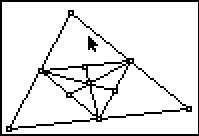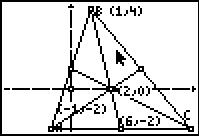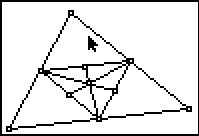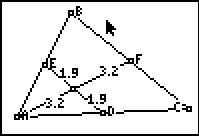# Activities

••• ##### Subject Area

• Math: Geometry: Analytic Geometry
• Math: Geometry: Triangles

• ##### Author9-12

45 Minutes

• ##### Device
• TI-83 Plus Family
• TI-84 Plus
• TI-84 Plus Silver Edition
•TI-84 Plus C Silver Edition
•TI-84 Plus CE
• ##### Software

TI Connect™
TI Connect™ CE

## Balancing Point#### Activity Overview

In this activity, students will explore the median and the centroid of a triangle.

#### Key Steps

•First, students will cut out a triangle and balance the triangle on their pencil.

•Students will then find the balancing point using the CabriTM Jr. application on their graphing calculator. They will discover that the medians of a triangle are concurrent and the balancing point occurs at the point of concurrency. The point of concurrency is the centroid.

•Students will extend the concept of the centroid by finding the centroid of the medial triangle. Students will discover that the original triangle and the medial triangle have the same centroid.